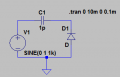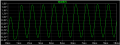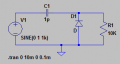# Why is the Output Voltage of a Positive Voltage Clamper with Resistive Load Different?

#### Beanz1114

Joined Nov 13, 2014
18
Hello there,
I have a quick question regarding the positive voltage clamper. I am well aware that they shift the AC signal by a certain DC level, which in my case is 1V. That is confirmed through my simulation in Figure 1. Figure 2 shows the output voltage, around 1.77V, taking into account the non-ideal turn on voltage of the diode which is around 0.23V. However, when a resistive load is connected to it, the output voltage is totally different than what I expected, and I can't, for the life of me figure out why. Figure 3 shows the circuit with resistive load and figure 4 shows the output voltage across the resistor. Shouldn't the output voltage still be the same as without the load as suggested by some of the websites (conceptelectronics and circuitstoday)?Figure 1: Positive Clamper CircuitFigure 2: Output Voltage of Positive Clamper taken across DiodeFigure 3 : Positive Voltage Clamper with Resistive LoadFigure 4 : Output Voltage across Rload

#### Dyslexicbloke

Joined Sep 4, 2010
565
The simple answer is that they are different circuits ...

look at the current in the diode/resistor and then consider the effect this has on the capacitor voltage, without the resistor how is the capacitor charge and discharge, its AC current, affected?

The beast way to learn is to play until you understand.

#### OBW0549

Joined Mar 2, 2015
3,566
However, when a resistive load is connected to it, the output voltage is totally different than what I expected, and I can't, for the life of me figure out why. Figure 3 shows the circuit with resistive load and figure 4 shows the output voltage across the resistor. Shouldn't the output voltage still be the same as without the load as suggested by some of the websites (conceptelectronics and circuitstoday)?
Look carefully at your circuit, in particular the value of capacitor C1. The reactance of a 1 pF capacitor at 1 kHz is Xc = 1/(2πfC) = 159 MΩ. This capacitive reactance, along with R1, sets up a voltage divider with a division ratio of 15900:1, giving an output voltage of 1 V/15900 or 63 μV, which is exactly what your simulation shows.

Change the value of C1 to 100 μF and see what happens.

•Beanz1114

#### crutschow

Joined Mar 14, 2008
31,529
Random part values lead to random results.#### RichardO

Joined May 4, 2013
2,270
Also, you need to pick a part number for the diode -- a 1N914 is a good choice to start with. Using the "default" parts can lead to very strange results in the simulation.

Thanks @OBW0549! I never thought of it as a voltage divider circuit before. That makes perfect sense. Thanks again!